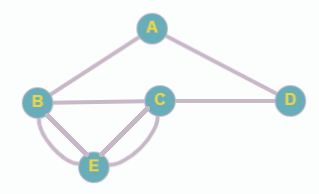# Parks in Exercises 23 and 24, a map of a park is shown with bridges connecting islands in a river to the banks. Represent the map as a graph. See Figures 5.3 and 5.4 on page 234. Is it possible to take a walk that crosses each bridge once and returns to the starting point without crossing any bridge twice? If not, can you do it if you do not end at the starting point? Explain how you know.### Mathematical Excursions (MindTap C...

4th Edition
Richard N. Aufmann + 3 others
Publisher: Cengage Learning
ISBN: 9781305965584

#### Solutions

Chapter
Section### Mathematical Excursions (MindTap C...

4th Edition
Richard N. Aufmann + 3 others
Publisher: Cengage Learning
ISBN: 9781305965584
Chapter 5.1, Problem 23ES
Textbook Problem
10 views

## Parks in Exercises 23 and 24, a map of a park is shown with bridges connecting islands in a river to the banks. Represent the map as a graph. See Figures 5.3 and 5.4 on page 234. Is it possible to take a walk that crosses each bridge once and returns to the starting point without crossing any bridge twice? If not, can you do it if you do not end at the starting point? Explain how you know.To determine

(a)

To graph:

To represent the given map as a graph.

### Explanation of Solution

Given information:

Given map of a park with bridges connecting islands in a river to the banks is

Calculation:

To represent the map as a graph, each land is considered a vertex and each bridge is considered an edge...

To determine

(b)

To determine if it is possible to take a walk that crosses each bridge once and returns to the starting point without crossing any bridge twice. If not, to check if the same is possible without ending at the starting point.

### Still sussing out bartleby?

Check out a sample textbook solution.

See a sample solution

#### The Solution to Your Study Problems

Bartleby provides explanations to thousands of textbook problems written by our experts, many with advanced degrees!

Get Started

Find more solutions based on key concepts
In problems 1-16, solve each equation. 15. Solve

Mathematical Applications for the Management, Life, and Social Sciences

Find the domain and range of each function. y=x+1 b. y={ x2,x0x,x0

Calculus: An Applied Approach (MindTap Course List)

Use the definition of the derivative to find the slope of the tangent line to the graph of the function f(x) = ...

Applied Calculus for the Managerial, Life, and Social Sciences: A Brief Approach

Sketch the graph of the function. 49. f(x)={|x|if|x|11if|x|1

Single Variable Calculus: Early Transcendentals, Volume I

Express each number in terms of j: 16

Elementary Technical Mathematics

Subtract 716332 .

Mathematics For Machine Technology

True or False: The absolute extrema of a continuous function on a closed interval always exist.

Study Guide for Stewart's Single Variable Calculus: Early Transcendentals, 8th

Find all real solutions of each equation. b+b+8=2

College Algebra (MindTap Course List)

When all the curves in a family G(x, y, c1) = 0 intersect orthogonally all the curves in another family H(x, y,...

A First Course in Differential Equations with Modeling Applications (MindTap Course List)

NCAA Basketball Game Scores. A sample of 10 NCAA college basketball game scores provided the following data. C...

Modern Business Statistics with Microsoft Office Excel (with XLSTAT Education Edition Printed Access Card) (MindTap Course List)

Reminder Round all answers to decimal places unless otherwise indicated. Hiking You are hiking in a hilly regio...

Functions and Change: A Modeling Approach to College Algebra (MindTap Course List)